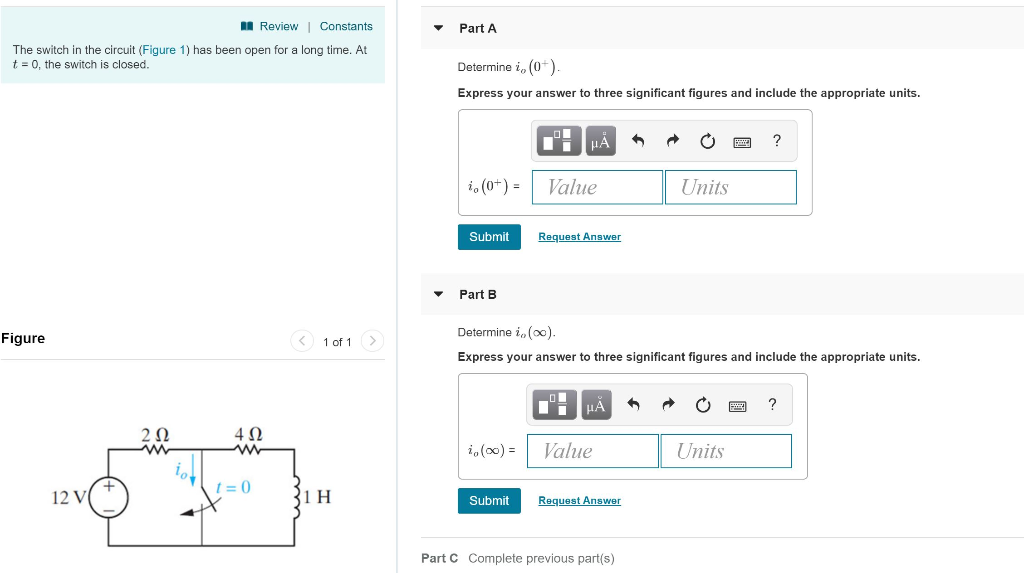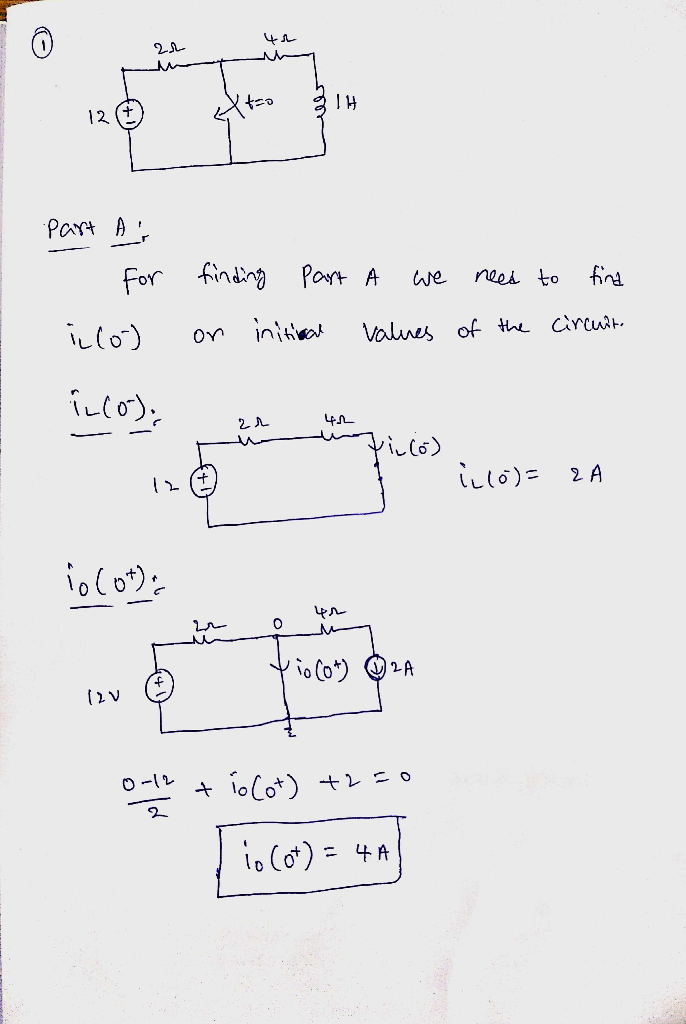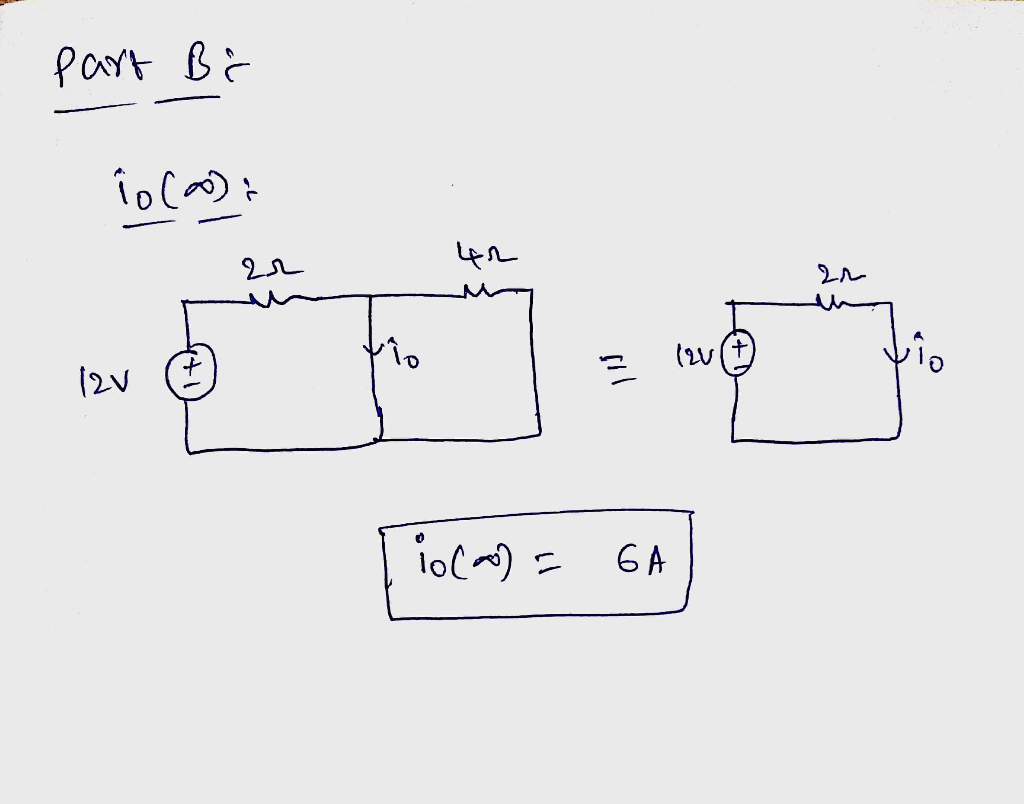# The switch in the circuit (figure 1) has been open for a long time. at t = 0 the switch is closed.ReviewConstants Part A The switch in the circuit (Figure 1) has been open for a long time. At t0, the switch is closed. Determine i, (0+) Express your answer to three significant figures and include the appropriate units. IA i (o+)Value Units Submit Request Answer Part B Determine in (oo) Figure < 1of1 Express your answer to three significant figures and include the appropriate units. 2Ω 4Ω (0)Value Units 12 V 1 H Submit Request Answer Part C Complete previous part(s)

For finding part A we need to find i_L (O^-) on initial values of the circuit i_L (O^-) i_L (O^-) = 2 A i_O (O^+) 0 – 12/2 + i_O (O^+) + 2 = 0 i_O (O^+) = 4 A rn part B: – I_O (infinity) i_O (infinity) = 6 A